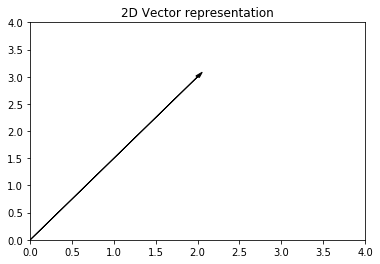Notebook

# Maths

In :
% run maths_functions.py


## Vectors

A vector, also refered to as a column matrix or a row matrix, is a matrix consisting or a single row or a single column of elements. For a vector with m elements, the size of a row vector would be $1\times{m}$, while for a column vector it would be $m \times 1$.

A vector can be represented in many ways, most common notations are an arrow or bar over the variable, or a bolded variable. It can be shown as the individual elements in a row or column. On plots, the vector is often shown as an arrow. 2-element vectors are often used in Cartesian/Complex 2D-space, while 3-element vectors are often used for 3D-space. 2D and 3D vectors are also called position vectors. For simplicity, vectors with three elements will be used.

$\vec{a}=\bar{a}=\begin{bmatrix} a_{1} \\ a_{2} \\ a_{3} \end{bmatrix} , \vec{b}=\bar{b}=\begin{bmatrix} b_{1} \\ b_{2} \\ b_{3} \end{bmatrix}$

In :
a = [2,3]
fig, ax = plt.subplots(1)
ax.set_xlim(0,4)
ax.set_ylim(0,4)
ax.set_title('2D Vector representation')
plt.show()When adding or subtracting vectors, each element is added or subtracted with its counterpart.

$\begin{bmatrix} a_1 \\ a_2 \\ a_3 \end{bmatrix} + \begin{bmatrix} b_1 \\ b_2 \\ b_3 \end{bmatrix} = \begin{bmatrix} a_1 + b_1 \\ a_2+b_2 \\ a_3+b_3 \end{bmatrix}$

An easy way to understand how it works is to align all vector arrows on a plot. Their sum is a vector that starts at the first vector's origin point and ends at the tip of the last vector.

#### Vector Magnitude

A vectors magnitude, also called aboslute value or norm. Norm of vector of a is denoted as $a$, $|\vec{a}|$ or $||\vec{a}||$. In the case of Euclidian space, 2D or 3D case, the magnitude is the square root of the sum of all elements. A vector with magnitude of 1 unit is called a unit vector and is denoted with a hat as $\hat{a}$.

$a = \sqrt{\sum_0^n a_n^2}$

#### Transposing

A transpose of a vector or matrix is a vector or matrix with the same values flipped over the diagonal. In the case of vectors, a row vector is the transpose of a column vector and vice versa. It is denoted with an upscript T ($^T$) or asterix (*).

$\begin{bmatrix} b_{1} \\ b_{2} \\ b_{3} \end{bmatrix}^T = \begin{bmatrix} b_{1} & b_{2} & b_{3}\end{bmatrix}$

#### Dot Product

Vectors can be multiplied in two different ways. Dot product is the elementwise multiplication and its result is a scalar number. In 3D vectors, it can also be computed as the product of the magnitutes of each vector multiplied by the cosine of the angle between them.

$\vec{a}.\vec{b} = a^Tb = a_1.b_1 + a_2.b_2 + a_3.b_3 = |{\vec{a}}|.|{\vec{b}}|.\cos{\theta}$

#### Cross Product

A cross product returns a vector that is perpendicular to both input vectors that has a magnitude equal to the product of the input magnitudes multiplied by the sine of the angle between the vectors. The side of the plane on which the perpendicular vector is, depends on the order of the input vectors.

$\vec{a}\times \vec{b} = \vec{a}\vec{b^T} = |a|.|b|.\sin(\theta).\hat{n}$, where $\hat{n}$ is a unit vector in the direction of the new vector.